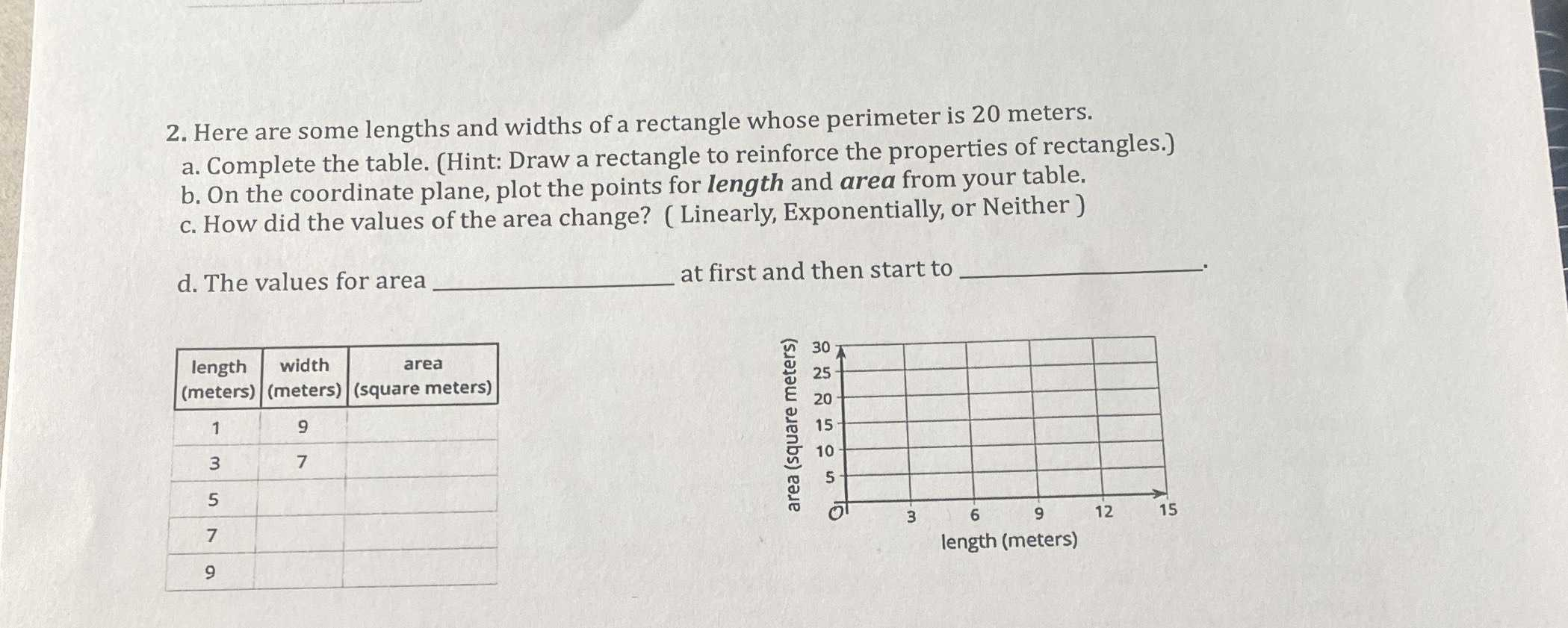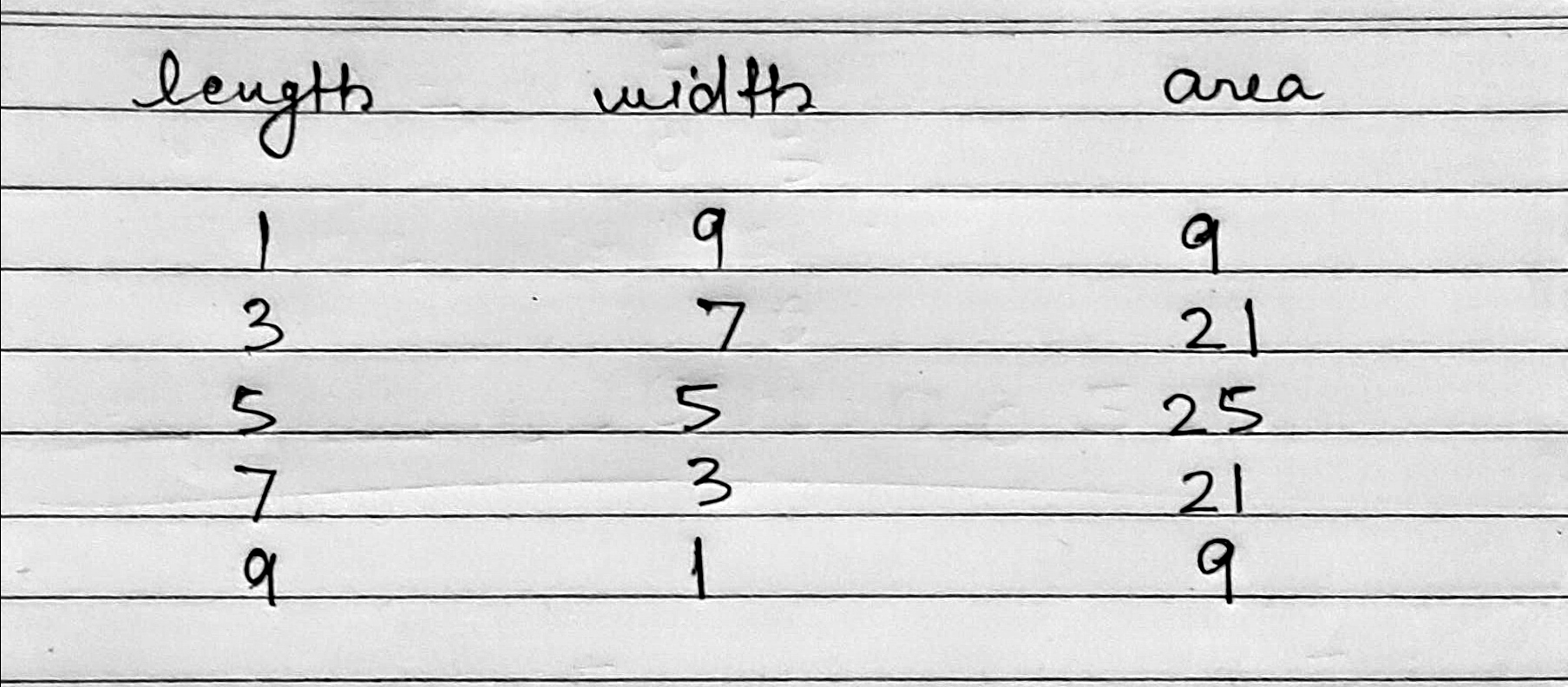### ¿Todavía tienes preguntas de matemáticas?

Pregunte a nuestros tutores expertos
Algebra
Pregunta2. Here are some lengths and widths of a rectangle whose perimeter is $$20$$ meters. a. Complete the table. (Hint: Draw a rectangle to reinforce the properties of rectangles.) b. On the coordinate plane, plot the points for length and area from your table. c. How did the values of the area change? (Linearly, Exponentially, or Neither) d. The values for area__ at first and then start to